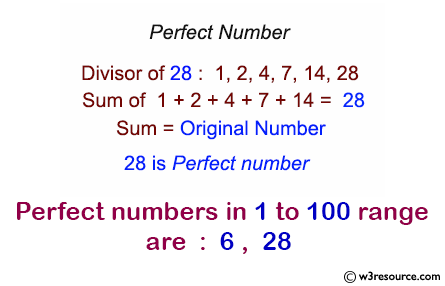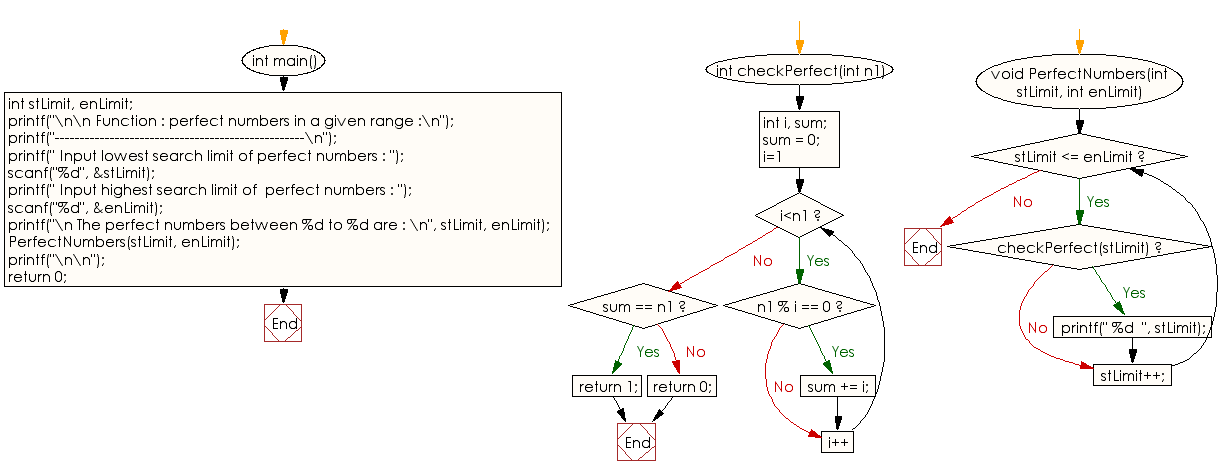﻿ C Program: Perfect numbers in a given range - w3resource

# C Exercises: Perfect numbers in a given range

## C Function : Exercise-10 with Solution

Write a program in C to print all perfect numbers in a given range using the function.

Pictorial Presentation:Sample Solution:

C Code:

``````#include <stdio.h>
/* Function declarations */
int checkPerfect(int n1);
void PerfectNumbers(int stLimit, int enLimit);

int main()
{
int stLimit, enLimit;
printf("\n\n Function : perfect numbers in a given range :\n");
printf("--------------------------------------------------\n");
printf(" Input lowest search limit of perfect numbers : ");
scanf("%d", &stLimit);
printf(" Input highest search limit of  perfect numbers : ");
scanf("%d", &enLimit);

printf("\n The perfect numbers between %d to %d are : \n", stLimit, enLimit);
PerfectNumbers(stLimit, enLimit);
printf("\n\n");
return 0;
}
// Checks whether the given number is perfect or not.

int checkPerfect(int n1)
{
int i, sum;

sum = 0;
for(i=1; i<n1; i++)
{
if(n1 % i == 0)
{
sum += i;
}
}
// If sum of proper positive divisors equals to given number
// then the number is perfect number
if(sum == n1)
return 1;
else
return 0;
}

void PerfectNumbers(int stLimit, int enLimit)
{
/* print perfect numbers from start to end */
while(stLimit <= enLimit)
{
if(checkPerfect(stLimit))
{
printf(" %d  ", stLimit);
}
stLimit++;
}
}
```
```

Sample Output:

``` Function : perfect numbers in a given range :
--------------------------------------------------
Input lowest search limit of perfect numbers : 1
Input highest search limit of  perfect numbers : 100

The perfect numbers between 1 to 100 are :
6   28
```

Flowchart:C Programming Code Editor:

What is the difficulty level of this exercise?

Test your Programming skills with w3resource's quiz.

﻿